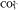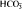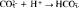# Solubility Product

The following article is from The Great Soviet Encyclopedia (1979). It might be outdated or ideologically biased.

## Solubility Product

the product of the ion concentrations in a saturated solution of a slightly soluble strong electrolyte. The exponents of the ion concentrations in the solubility product are equal to the corresponding ion coefficients in the equation of electrolyte dissociation. For nonideal solutions, the concentrations are replaced by activities, and the product thus obtained is called the activity product. There is a characteristic value of the solubility product for each electrolyte at a given temperature in a given solvent.

The constancy of the solubility product is derived from the law of mass action. It represents a specific application of this law to the case of the equilibrium between an electrolyte in the solid phase and a saturated solution of the same electrolyte. It is assumed that the electrolyte in the solution is fully dissociated. The solubility product is most accurately measured by the electromotive force (emf) method; another common method of measurement derives from the determination of solubility by the electrical conductivity of saturated solutions. The solubility product for many compounds is established with sufficient accuracy for all practical purposes. In tables, the solubility product is usually given for a temperature of 25°C (sometimes for 18°C).

Since the solubility product is a constant, it follows that if the product of the ion concentrations in a solution exceeds the solubility product’s value, precipitation will occur. If the opposite is true, no precipitate is formed. This consequence permits a control of the ion content in a solution by means of precipitation, dissolving, and salting-out—all key processes in analytical chemistry and chemical technology. When, for example, the concentration of one ion is increased by introducing a new electrolyte with the same type of cation or anión into the solution, the concentration of the other ion is decreased by the precipitation of part of the poorly soluble electrolyte. The solubility decreases only to a certain minimum value, after which an increase in solubility may be observed owing to either the formation of complex ions or the increase in the ionic strength of the solution.

An increase in solubility may be achieved by binding one ion in the solution so as to form another ion that does not yield a slightly soluble compound. For example, in order to dissolve a CaCO3 precipitate, theion is bound with the aid of a H+ ion into a weakly dissociatedion:. The concentration ofions is thereby decreased, and the precipitate will dissolve until the solubility product is achieved.

### REFERENCES

Spravochnik khimika, 2nd ed., vol. 3. Moscow-Leningrad, 1965.
Kursfizicheskoi khimii, 2nd ed., vol. 2. Edited by Ia. I. Gerasimov. Moscow, 1973. Chapter 18.

N. F. STEPANOV

Mentioned in ?
References in periodicals archive ?
According to Zumdahl , the aqueous solubility of sparingly soluble compounds in equilibrium with the solid phase is constant and corresponds to the value of the solubility product constant [K.sub.sp].
The aqueous [Cd.sup.2+], P[O.sub.4.sup.3-], and [F.sup.-] activities in the final equilibrated solution (5040 h, 5760 h, and 7200 h) were computed with the program PHREEQC to estimate the solubility product of the synthetic cadmium fluorapatite [[Cd.sub.5][(P[O.sub.4]).sub.3]F].
The two common factors involved are high levels of parathyroid hormone and a remarkable increase in the calcium-phosphate solubility product; however, calcification can also be seen without any of these two factors.
The implication is that when TSP/MAP/DAP fertilisers containing the maleic-itaconic acid copolymer are applied to alkaline soil, binding of P by exchangeable Ca or free CaC[O.sub.3] to form DCPD precipitation will always take place until soil solution P concentration is in equilibrium with the solubility product constant for DCPD before the remaining Ca is chelated with the copolymer even the amount of the copolymer used is very high.
For example the [k.sub.sp] (ionic solubility product) for calcite at 0 and 50[degrees]C are [10.sup.-8.02] and [10.sup.-8.63] respectively .
The ninth edition adds an experiment on determining the solubility product of barium iodate and an introduction to Excel in the appendices.
The solubility of CaS[O.sub.4] in water is given by the solubility product constant ([K.sub.sp]: 4.93 x [10.sup.-5] [M.sup.2]; Lide, 2004; though there is some small variation in published values).
Thus, investigations were performed using a solubility product approach to evaluate how the effects of sulfur and manganese on gray iron properties are related to the dissolved amounts of both elements remaining in the molten iron when solidification begins.
Bennett AC, Adams F (1972) Solubility and solubility product of gypsum in soil solutions and other aqueous solutions.
The thermodynamic solubility product of the nano HA was 3.3 X [10.sup.-94] compared to 1 X [10.sup.-117] for macro scale crystalline HA.

Site: Follow: Share:
Open / Close